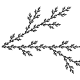You are currently browsing the tag archive for the ‘coboundaries’ tag.

A dynamical system is a space X, together with an action$(g,x) \mapsto gx$ of some group$G = (G,\cdot)$.  [In practice, one often places topological or measure-theoretic structure on X or G, but this will not be relevant for the current discussion.  In most applications, G is an abelian (additive) group such as the integers${\Bbb Z}$ or the reals${\Bbb R}$, but I prefer to use multiplicative notation here.]  A useful notion in the subject is that of an (abelian) cocycle; this is a function$\rho: G \times X \to U$ taking values in an abelian group$U = (U,+)$ that obeys the cocycle equation$\rho(gh, x) = \rho(h,x) + \rho(g,hx)$ (1)

for all$g,h \in G$ and$x \in X$.  [Again, if one is placing topological or measure-theoretic structure on the system, one would want$\rho$ to be continuous or measurable, but we will ignore these issues.] The significance of cocycles in the subject is that they allow one to construct (abelian) extensions or skew products$X \times_\rho U$ of the original dynamical system X, defined as the Cartesian product$\{ (x,u): x \in X, u \in U \}$ with the group action$g(x,u) := (gx,u + \rho(g,x))$.  (The cocycle equation (1) is needed to ensure that one indeed has a group action, and in particular that$(gh)(x,u) = g(h(x,u))$.)  This turns out to be a useful means to build complex dynamical systems out of simpler ones.  (For instance, one can build nilsystems by starting with a point and taking a finite number of abelian extensions of that point by a certain type of cocycle.)

A special type of cocycle is a coboundary; this is a cocycle$\rho: G \times X \to U$ that takes the form$\rho(g,x) := F(gx) - F(x)$ for some function$F: X \to U$.  (Note that the cocycle equation (1) is automaticaly satisfied if$\rho$ is of this form.)  An extension$X \times_\rho U$ of a dynamical system by a coboundary$\rho(g,x) := F(gx) - F(x)$ can be conjugated to the trivial extension$X \times_0 U$ by the change of variables$(x,u) \mapsto (x,u-F(x))$.

While every coboundary is a cocycle, the converse is not always true.  (For instance, if X is a point, the only coboundary is the zero function, whereas a cocycle is essentially the same thing as a homomorphism from G to U, so in many cases there will be more cocycles than coboundaries.  For a contrasting example, if X and G are finite (for simplicity) and G acts freely on X, it is not difficult to see that every cocycle is a coboundary.)  One can measure the extent to which this converse fails by introducing the first cohomology group$H^1(G,X,U) := Z^1(G,X,U) / B^1(G,X,U)$, where$Z^1(G,X,U)$ is the space of cocycles$\rho: G \times X \to U$ and$B^1(G,X,U)$ is the space of coboundaries (note that both spaces are abelian groups).  In my forthcoming paper with Vitaly Bergelson and Tamar Ziegler on the ergodic inverse Gowers conjecture (which should be available shortly), we make substantial use of some basic facts about this cohomology group (in the category of measure-preserving systems) that were established in a paper of Host and Kra.

The above terminology of cocycles, coboundaries, and cohomology groups of course comes from the theory of cohomology in algebraic topology.  Comparing the formal definitions of cohomology groups in that theory with the ones given above, there is certainly quite a bit of similarity, but in the dynamical systems literature the precise connection does not seem to be heavily emphasised.   The purpose of this post is to record the precise fashion in which dynamical systems cohomology is a special case of cochain complex cohomology from algebraic topology, and more specifically is analogous to singular cohomology (and can also be viewed as the group cohomology of the space of scalar-valued functions on X, when viewed as a G-module); this is not particularly difficult, but I found it an instructive exercise (especially given that my algebraic topology is extremely rusty), though perhaps this post is more for my own benefit that for anyone else.Usman Nizami on AppletsAnonymous on 247B, Notes 3: pseudodifferent…Xiaoyan Su on 247B, Notes 3: pseudodifferent…Rex on 247B, Notes 4: almost everywhe…Rex on 247B, Notes 4: almost everywhe…Terence Tao on 254A, Notes 1: Local well-pose…Terence Tao on The completeness and compactne…Terence Tao on 247B, Notes 3: pseudodifferent…Terence Tao on 247B, Notes 4: almost everywhe…John Mangual on 247B, Notes 4: almost everywhe…Liam Shakib Llamazar… on 254A, Notes 1: Local well-pose…Alan Chang on 247B, Notes 4: almost everywhe…Anonymous on 247B, Notes 1: Restriction…Anonymous on 247B, Notes 4: almost everywhe…Anonymous on 247B, Notes 1: Restriction…